Topic 14.8: Higher-Order Initial-Value Problems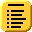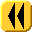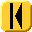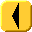Introduction Notes Theory HOWTO Examples Engineering Error Questions Matlab Maple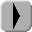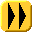We have worked with 1st-order initial-value problems. In this topic, we discuss how we can convert an nth-order initial-value problem (an nth-order differential equation and n initial values) into a system of n 1st-order initial-value problems.

Background

Useful background for this topic includes:

References

• Bradie, Section 7.8, Systems of Equations and Higher-Order Equations, p.628.
• Mathews, Section 9.7, Systems of Differential Equations, p.521.